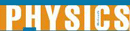# Momentum and Newton's Second Law - Research Article from World of Physics

This encyclopedia article consists of approximately 2 pages of information about Momentum and Newton's Second Law.
 This section contains 573 words (approx. 2 pages at 300 words per page) View a FREE sampleEnglish physicist and mathematician Sir Isaac Newton's second law, usually written as F = ma where F is the force, m is the mass of the object and a is its acceleration, states that the acceleration of a body is proportional to the force applied to it and inversely proportional to its mass. Although the second law is usually written in this form it is not the form that Newton originally used in his Philosophiae Naturalis Principia Mathematica (Mathematical Principles of Natural Philosophy) nor can it be used in situations where the mass of the object may be changing. The original form was written in terms of momentum and is currently expressed as F = dp/dt where F is the force, dp is the change in momentum and dt is the time interval. Although Newton expressed his second law of motion...

(read more)

 This section contains 573 words (approx. 2 pages at 300 words per page) View a FREE sampleMore summaries and resources for teaching or studying Momentum and Newton's Second Law.
CopyrightsMomentum and Newton's Second Law from Gale. ©2005-2006 Thomson Gale, a part of the Thomson Corporation. All rights reserved.